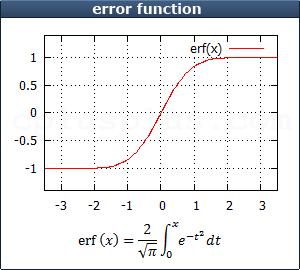function
<cmath> <ctgmath>

# erf

```     double erf  (double x);
float erff (float x);
long double erfl (long double x);
```
```     double erf (double x);
float erf (float x);
long double erf (long double x);
Compute error functionReturns the error function value for x.

Header <tgmath.h> provides a type-generic macro version of this function.
Additional overloads are provided in this header (`<cmath>`) for the integral types: These overloads effectively cast x to a `double` before calculations (defined for T being any integral type).

### Parameters

x
Parameter for the error function.

### Return Value

Error function value for x.

### Example

 ``123456789101112`` ``````/* erf example */ #include /* printf */ #include /* erf */ int main () { double param, result; param = 1.0; result = erf (param); printf ("erf (%f) = %f\n", param, result ); return 0; }``````

Output:
 ``` erf (1.000000) = 0.842701 ```